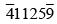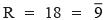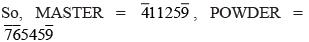Courses

# Olympiad Test: Coding – Decoding - 2

## 20 Questions MCQ Test Science Olympiad Class 6 | Olympiad Test: Coding – Decoding - 2

Description
This mock test of Olympiad Test: Coding – Decoding - 2 for Class 6 helps you for every Class 6 entrance exam. This contains 20 Multiple Choice Questions for Class 6 Olympiad Test: Coding – Decoding - 2 (mcq) to study with solutions a complete question bank. The solved questions answers in this Olympiad Test: Coding – Decoding - 2 quiz give you a good mix of easy questions and tough questions. Class 6 students definitely take this Olympiad Test: Coding – Decoding - 2 exercise for a better result in the exam. You can find other Olympiad Test: Coding – Decoding - 2 extra questions, long questions & short questions for Class 6 on EduRev as well by searching above.
QUESTION: 1

### If CONCEPT is written as unmulqr and FRIEND is written as ysglmt, then how is PREDICT written in that code?

Solution:

Letter : C O N E P T F R I D
Code : u n m l q r y s g t
The code for PREDICT is qsltgur.

QUESTION: 2

### In a certain code, FIRE is written as QHOE and MOVE as ZMWE. Following the same rule of coding what should be the code for the word OVER?

Solution:

Letter: F I R E M O V
Code: Q H O E Z M W
The code for OVER is MWEO.

QUESTION: 3

### If WORK is coded as 4 – 12 – 9 – 16, then how will you code WOMAN?

Solution:

Each letter is coded by the numeral obtained by subtracting from 27 then numeral denoting the position of the letter in the English alphabet. W, O, M, A, N are 23rd, 15th, 13th, 1st and 14th letters. So their codes are (27 – 23), (27 – 15), (27 – 13), (27 – 1), (27 – 14)
i.e. 4, 12, 14, 26, 13 respectively.

QUESTION: 4

If GO = 32, SHE = 49, then SOME will be equal to

Solution:

In the given code, Z = 1, Y = 2, X = 3, ……. C = 24, B = 25, Z = 26.
So, GO = 20 + 12 = 32 and SHE = 8 + 19 + 22 = 49.
Similarly, SOME = S + O + M + E = 8 + 12 + 14 + 22 = 56.

QUESTION: 5

If ZIP = 198 and ZAP = 246, then how will you code VIP?

Solution:

Taking Z = 2, Y = 3, …., N = 14, ……, B = 26,
A = 27
ZIP
= (Z + I + P) × 6 = (2 + 19 + 12) × 6 = 33 × 6
= 198
VIP
= (V + I + P) × 6 = (6 + 19 + 12) × 6 = 37 × 6
= 222

QUESTION: 6

If MASTER is coded as, then POWDER will be coded as.

Solution:

Let A = 1, B = 2, C = 3, ……, Z = 26. Now, M = 13 = 4 (Remainder obtained after dividing by 9).
S = 19 = 1 (Remainder obtained after dividing by 9 twice)
T = 20 = 2 (Remainder obtained after dividing by 9 twice)(Remainder obtained after dividing by 9)QUESTION: 7

In a certain code, BRAIN is written as * % ÷ # × and TIER is written as \$ # + %. How is RENT written in that code?

Solution:

Letter : B R A I N T E
Code : ∗  % ÷ # × \$ +
The code for RENT is % + × \$

QUESTION: 8

If DELHI is coded as 73541 and CALCUTTA as 82589662, how can CALICUT be coded?

Solution:

Letter: D E L H I C A U T
Code: 7 3 5 4 1 8 2 9 6
The code for CALICUT is 8251896.

QUESTION: 9

If NOIDA is written as 39658, how will INDIA be written?

Solution:

Letter : N O I D A
Code : 3 9 6 5 8
The code for INDIA is 63568.

QUESTION: 10

If ENGLAND is written as 1234526 and FRANCE is written as 785291, how is GREECE coded?

Solution:

Letter: E N G L A D F R C
Code: 1 2 3 4 5 6 7 8 9
The code for GREECE is 381191.

QUESTION: 11

If REQUEST is written as S2R52TU, then how will ACID be written?

Solution:

Vowels A, E, I, O, U are coded as 1, 2, 3, 4, 5 respectively. Each of the consonants in the word is moved one step forward to give the corresponding letter of the code so, the code for ACID becomes ID3E.

QUESTION: 12

In a certain code, DESK is written as #. 52, RIDE is written as % 7# . how is RISK written in that code?

Solution:

Letter: D E S K R I
Code: # 5 2 % 7
The code for RISK is % 752.

QUESTION: 13

In a certain code, EAT is written as 318 and CHAIR is written as 24156. What will TEACHER be written as?

Solution:

Letter: E A T C H I R
Code: 3 1 8 2 4 5 6
The code for TEACHER is 8312436.

QUESTION: 14

In a certain code, DEAF is written as 3587 and FILE is written as 7465. How is IDEAL written in that code?

Solution:

Letter: D E A F I L
Code: 3 5 8 7 4 6
The code for IDEAL is 43586.

QUESTION: 15

If DRIVER = 12, PEDESTRIAN = 20, ACCIDENT = 16, then CAR = ?

Solution:

CAR = (Number of letters in CAR) × 2 = 3 × 2 = 6

QUESTION: 16

If ‘Oranges’ are ‘apples’, ‘bananas’ are ‘apricots’, ‘apples’ are ‘chillies’, ‘apricots’ are ‘oranges’ and chillies are ‘bananas’, then which of the following are green in colour?

Solution:

‘Chillies’ are green in colour and as given, ‘chillies’ are ‘bananas’. So, ‘bananas’ are green in colour.

QUESTION: 17

If ‘sky’ is ‘star ’, ‘star ’ is ‘cloud’, ‘cloud’ is earth, ‘earth’ is ‘tree’ and ‘tree’ is ‘book’, then where do the birds fly?

Solution:

Birds fly in the ‘sky’ and as given, ‘sky’ is ‘star’. So, birds fly in the ‘star’.

QUESTION: 18

If ‘blue’ means ‘green’, ‘green’ means ‘white’, ‘white’ means ‘yellow’ means ‘black’, ‘black’ means ‘red’ and ‘red’ means ‘brown’, then what is the colour of milk?

Solution:

The colour of milk is ‘white’. But, as given ‘green’ means ‘white’. So, the colour of milk is green.

QUESTION: 19

If ‘eraser ’ is called ‘box’, ‘box’ is called ‘pencil’, ‘pencil’ is called ‘sharpener’ and ‘sharpener’ is called ‘bag’, what will a child write with?

Solution:

A child will write with a ‘pencil’ and ‘pencil’ is called sharpener. So, a child will write with a ‘sharpener’.

QUESTION: 20

If ‘dust’ is called ‘air ’, ‘air ’ is called ‘fire’, ‘fire’ is called ‘water’, ‘water’ is called ‘colour’, ‘colour’ is called ‘rain’ and ‘rain’ is called ‘dust’, then where do fish live?

Solution:

Fishes l ive in ‘water’ and as given, ‘water’ is called ‘colour’. So, fishes live in ‘colour’.